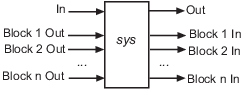Documentation

linlft

Linearize model while removing contribution of specified blocks

Syntax

lin_fixed = linlft(sys,io,blocks)
[lin_fixed,lin_blocks] = linlft(___)
[___] = linlft(___,opt)

Description

lin_fixed = linlft(sys,io,blocks) linearizes the Simulink® model named sys while removing the contribution of certain blocks. Specify sys as a character vector or string. Specify the full block path of the blocks to ignore in the cell array of character vectors or string array called blocks. The linearization occurs at the operating point specified in the Simulink model, which includes the ignored blocks. You can optionally specify linearization points (linear analysis points) in the I/O object io. The resulting linear model lin_fixed has this form:The top channels In and Out correspond to the linearization points you specify in the I/O object io. The remaining channels correspond to the connection to the ignored blocks.

When you use linlft and specify the 'block-by-block' linearization algorithm in linearizeOptions, you can use all the variations of the input arguments for linearize.

You can linearize the ignored blocks separately using linearize, and then combine the linearization results using linlftfold.

[lin_fixed,lin_blocks] = linlft(___) returns the linearizations for each of the blocks specified in blocks. If blocks contains a single block path, lin_blocks is a single state-space (ss) model. If blocks is an array identifying multiple blocks, lin_blocks is a cell array of state-space models. The full block path for each block in lin_blocks is stored in the Notes property of the state-space model.

[___] = linlft(___,opt) uses additional linearization options, specified as a linearizeOptions option set.

Examples

Linearize the following parts of the scdtopmdl Simulink model separately, and then combine the results:

• Fixed portion, which contains everything except the Parameter Varying Controller model reference• Parameter Varying Controller model reference, which references the scdrefmdl model% Open the Simulink model
topmdl = 'scdtopmdl';

% Linearize the model without the Parameter Varying Controller
io = getlinio(topmdl);
blocks = {'scdtopmdl/Parameter Varying Controller'};
sys_fixed = linlft(topmdl,io,blocks);

% Linearize the Parameter Varying Controller
refmdl = 'scdrefmdl';
sys_pv = linearize(refmdl);

% Combine the results
BlockSubs(1) = struct('Name',blocks{1},'Value',sys_pv);
sys_fold = linlftfold(sys_fixed,BlockSubs);